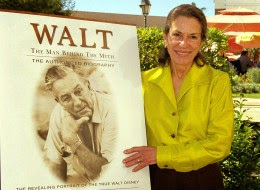## Sunday, April 13, 2014

### 33 42 Watch | Diane Disney's death on the premiere of 'Frozen', murdered by the numbers?Diane Disney-Miller was murdered by the 33

• Walt = 5+1+3+2 = 11
• Disney = 4+9+1+5+5+7 = 31
• Walt Disney = 42
• Freemason = 6+9+5+5+4+1+1+6+5 = 42
Diane Disney-Miller is the only child of Walt Disney, and his wife, Lillian Bounds Disney.  Diane was born on December 18, 1933; and it probably wasn't by coincidence that she was born in the year '33.  Let us examine further.
• Diane = 4+9+1+14+5 = 33
• Disney = 4+9+19+14+5+25 = 76
• Miller = 13+9+12+12+5+18 = 69
• Diane Disney Miller = 178
• Diane = 4+9+1+5+5 = 24
• Disney = 4+9+1+5+5+7 = 31
• Miller = 4+9+3+3+5+9 = 33
• Disney on 33rd Parallel in Anaheim (Orange County)
• Anaheim = 1+5+1+8+5+9+4 = 33
• Orange = 6+9+1+5+7+5 = 33
• Diane Disney-Miller = 88
After becoming married, she took on the last name Disney-Miller.  The name creates the ranging degrees of Scottish Rite Freemasonry, 31-33.  Her initials are also interesting, 444, connecting to 'Masonic' in English Gematria.
• Miller = 4+9+3+3+5+9 = 33 (Pythagorean)
• Miller = 13+9+12+12+5+18 = 69
• Diane Disney-Miller = DDM = 444
Diane's mother was Lillian Bounds Disney.
• Lillian = 3+9+3+3+9+1+5 = 33 (Pythagorean)
• Lillian =12+9+12+12+9+1+14 = 69
• Bounds = 2+6+3+5+4+1 = 21 (Pythagorean)
Doesn't that seem like a lot of 33?  It reminds me of the Kennedy family.
• Kennedy = 2+5+5+5+5+4+7 = 33
Then there is also Walter Elias Disney, better known as "Walt", himself.  He was born December 5, 1905 and died December 15, 1966.
• Disney = 4+9+1+5+5+7 = 31
• 12/15/1966 = 1+2+1+5+1+9+6+6 = 31
• 31 is the first degree of Freemasonry, running 31 through 33
• 1966 was the year the Church of Satan was established in San Francisco, California
Walt Disney is known for the movies he made, and the theme park he helped create, Disneyland, located directly on the 33rd Parallel North.  If you're an adult and visiting Disneyland, you might notice the only place you can drink at the entire resort is called "Club 33".
• Disneyland = 4+9+1+5+5+7+3+1+5+4 = 44
DDM = 444
While Walt Disney's death is suspicious in terms of numerology, Diane Disney-Miller's death makes his look like child's play.  Before we get into that, first some back story on the Freemasons.  The number 33 is extremely important to religions of the world as well as the secret society for Freemasons, which many of the founders of the United States were a part of.  As stated above, they have a ranging degree of 31 to 33, and have coded these numbers into everything from the adoption of the flag, to the establishing of the first state Delaware, to even the name of Delaware.
• Delaware was established December 7, 1787
• 12/7/1787 = 1+2+7+1+7+8+7 = 33
• Delaware = 5+4+3+1+5+1+9+5 = 33
• The flag was adopted June 14, 1777
• 6/14/1777 = 6+1+4+1+7+7+7 = 33
Long before the United States, and even before the Freemasons, were the Knights of Templar, who were founded in 1119, and later gave birth to what is known as Freemasonry.  This past November 19, 2013, or 11/19/13, Diane Disney-Miller was found dead after "sustaining a fall"...  Maybe so, but I doubt it.  I think a "friend" paid her a visit in the same way a "friend" paid Walt Disney a visit on December 15, 1966.  When it comes to the 33, a "hike" can be quite dangerous.
• Hike = 8+9+11+5 = 33 (Diane) (Number of Sacrifice)
• Knight = 2+5+9+7+8+2 = 33
• 12/18/1933 = 12+18+19+33 = 82 (Armageddon)
• 12/18/1933 = 12+18+(1+9+3+3) = 46 (Sacrifice)
• 12/18/1933 = 1+2+1+8+1+9+3+3 = 28
• 12/18/33 = 12+18+33 = 62 (Mason)
• 11/19/2016 = 11+19+20+16 = 66
• 11/19/2016 = 11+19+(2+0+1+6) = 39 (Frozen)
• 11/19/2016 = 1+1+1+9+2+0+1+6 = 21
• 11/19/16 = 11+19+16 = 46 (Sacrifice)
If you doubt Disney synchs "things" up with dates, check out the movie The Lost Treasure, starring Nicholas Cage, which is about the Knights of Templar and Freemasons and was released November 19, 2004; 9 years before the death of Walt Disney's only daughter.

Imagine if the next staged hoax of a major terrorist attack took place at Disneyland; it would probably be the most heartbreaking event imaginable for the many millions of Disney fans living in the United States; the people who have been tricked into supporting a company that is more evil than just about any other, which exploits its laborers and subconsciously attacks the minds of innocent children with its media programming.  If you doubt that, go back and watch the Disney films you saw as a kid and see what you now pick up on as an adult... it is astonishing.  Almost as astonishing as all the 33s in the names of the wife and child of Walt Disney.

When I think of Disney, I think of the year 2007 and working in Seattle with at-risk youth, where the only radio programming they could listen to was "Radio Disney" where at the time there was a song about being "suicidal, suicidal..." and all the kids were singing it.  Trusting in Disney is like trusting in Satan, which makes perfect sense, because Satan is always the best salesman.

PS  When I use the word Satan, I'm referring to what is wrong with the world, the temptations that we collectively give into and sustain things as they are, good and bad.  Disney movies are part of this.